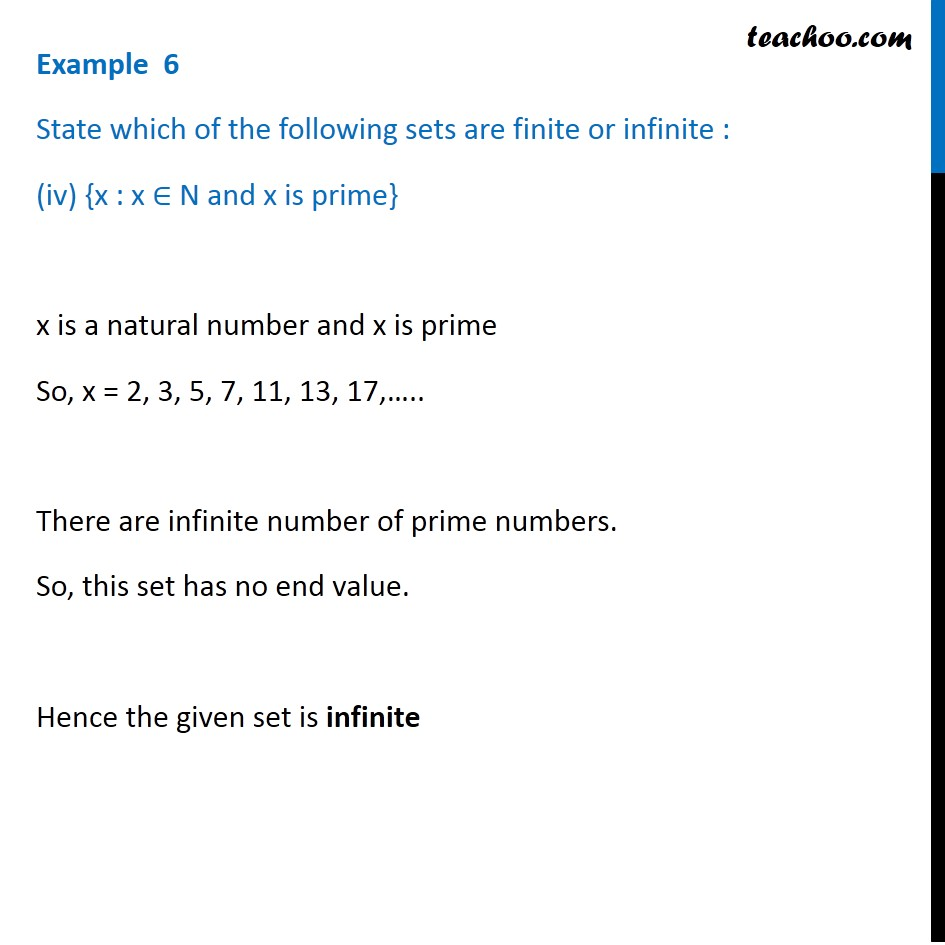Examples

Chapter 1 Class 11 Sets
Serial order wiseLearn in your speed, with individual attention - Teachoo Maths 1-on-1 Class

### Transcript

Example 6 State which of the following sets are finite or infinite : (iv) {x : x ∈ N and x is prime} x is a natural number and x is prime So, x = 2, 3, 5, 7, 11, 13, 17,….. There are infinite number of prime numbers. So, this set has no end value. Hence the given set is infinite